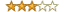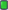Thread: nvml debug log decrypt View Single Post
#1
 winndyVIPJoin Date: Sep 2005 Posts: 232 Rept. Given: 105 Rept. Rcvd 25 Times in 11 Posts Thanks Given: 23 Thanks Rcvd at 16 Times in 13 Postsnvml debug log decrypt

Just for fun:

__NVML_DBG_FILE=./nvml.log __NVML_DBG_LVL=DEBUG ./sample_nvml

Code:
```static uint32_t x = 0, y = 0, z = 0,
w = 0, v = 0, d = 0;

void xorwow_init_seed()
{
x = 0x0D3DAECB8, y = 0x1D4D4848, z = 0x0AA7B8E81,
w = 0x23CC0EC3, v = 0x7645F3ED, d = 0x0E44A4F49;
}

void xorwow_encrypt(char* buffer, int len)
{
int i = 0;
for(i=0 ; i < len; i++){
uint32_t t = (x^(x>>2)); x = y; y = z; z = w; w = v; v = (v^(v<<4))^(t^(t<<1));
uint32_t r = ((d+=362437)+v);
buffer[i] += (r & 0xFF);
}
return;
}```